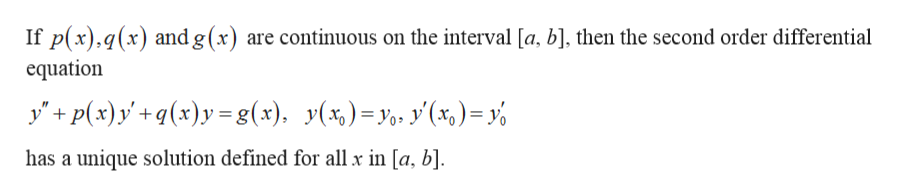# Using the existence and uniqueness theorem for second order linear ordinary differential equations, find the largest interval in which the solution to the initial value is certain to exist.t(t2-4)y"-ty'+3t2y=0, y(1)=1, y'(1)=3

Question
15 views

Using the existence and uniqueness theorem for second order linear ordinary differential equations, find the largest interval in which the solution to the initial value is certain to exist.

t(t2-4)y"-ty'+3t2y=0, y(1)=1, y'(1)=3

check_circle

Step 1

The given initial value problem is:

Step 2

Existence and Uniqueness Theorem:help_outlineImage TranscriptioncloseIf p(x),q(x) and g(x) are continuous on the interval [a, b], then the second order differential equation y'p(x)xy=g(x), y(x)y, y(x,)= has a unique solution defined for all x in [a, b]. fullscreen
Step 3

Redefine the differential e...

### Want to see the full answer?

See Solution

#### Want to see this answer and more?

Solutions are written by subject experts who are available 24/7. Questions are typically answered within 1 hour.*

See Solution
*Response times may vary by subject and question.
Tagged in

### Math